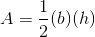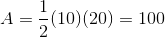# ACT Math : How to find the area of an acute / obtuse isosceles triangle

## Example Questions

### Example Question #212 : Plane Geometry

What is the area of an isosceles triangle with a vertex ofdegrees and two sides equal to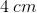?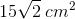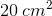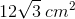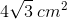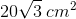Explanation:

Based on the description of your triangle, you can draw the following figure: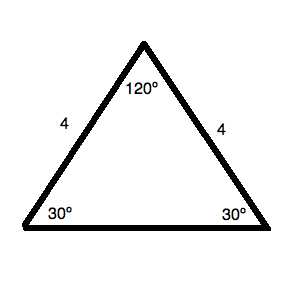You can do this because you know:

1. The two equivalent sides are given.
2. Since a triangle isdegrees, you have only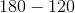ordegrees left for the two angles of equal size. Therefore, those two angles must bedegrees anddegrees.

Now, based on the properties of an isosceles triangle, you can draw the following as well: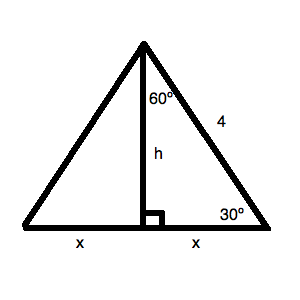Based on your standard reference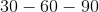triangle, you know: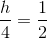Therefore,is.

This means thatis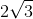and the total base of the triangle is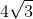.

Now, the area of the triangle is: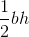or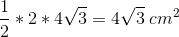### Example Question #213 : Plane Geometry

An isosceles triangle has a height ofand a base of. What is its area?Explanation:

Use the formula for area of a triangle: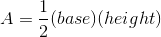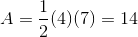### Example Question #214 : Plane Geometry

An isosceles triangle has a base length ofand a height that is twice its base length. What is the area of this triangle?Explanation:

1. Find the height of the triangle: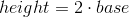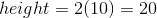2. Use the formula for area of a triangle: